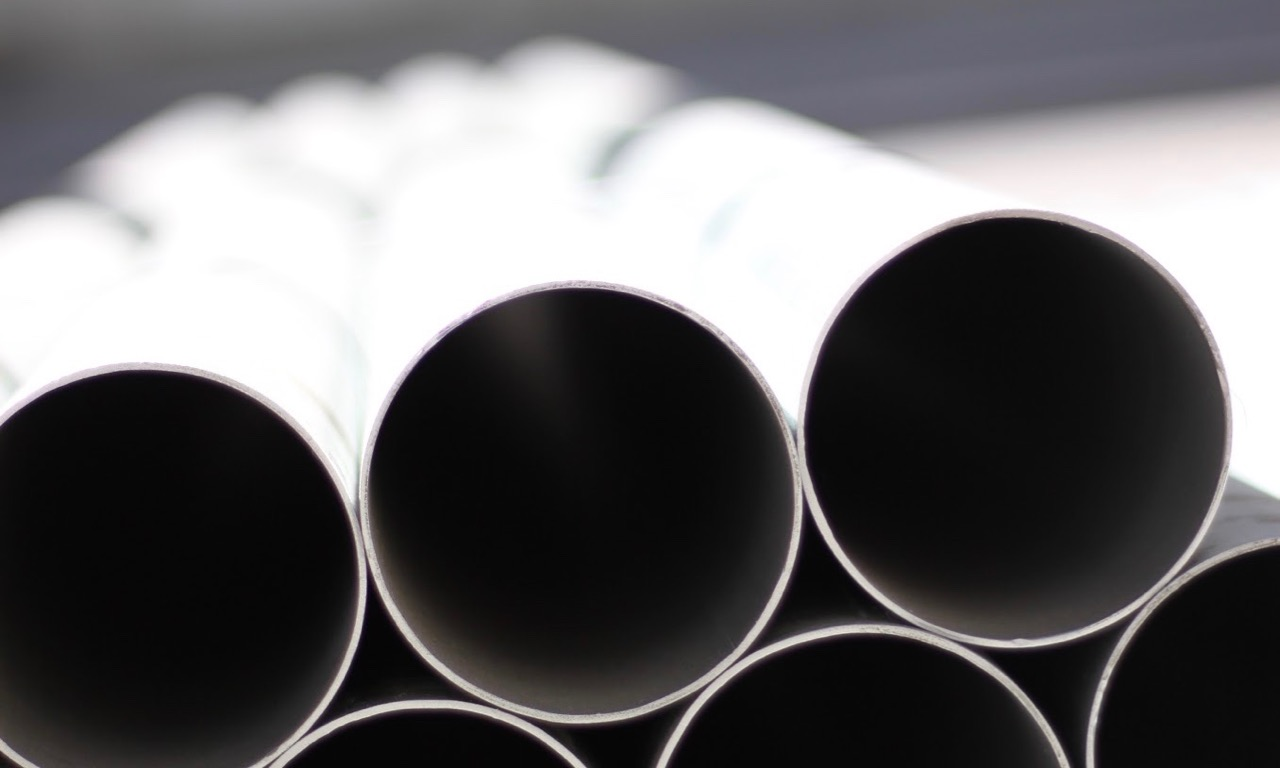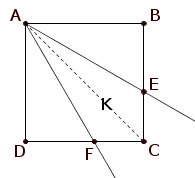## ________________

Last week’s practice focused on squares, so Coach Newton declares that this week is dedicated to circles.

## ________________### Circle Stack

Two cylinders 80 centimeters long — one with a radius of 17 centimeters, the other with a radius of 9 centimeters — are packed tightly into the shallowest possible box that is 50 centimeters wide and 80 centimeters long.

How deep is the box?### Three Circles, One Distance

In the diagram, circle c has radius 3, and A and B are any distinct points on c with less than 90 degrees of arc between them. Circle a has center A and goes through B, while b has center B and goes through A. Point E is the intersection of a and b inside c, and F is the other intersection point of a and c. Point D is the other intersection of line FE with c.

What is the distance from D to E? If you’re stuck, Coach Newton’s advice is to remember that when a problem leaves something arbitrary, choose it to make things as easy as possible. Can you find a good angle for arc AB? Maybe one that makes DE and EF equal?

## Solutions to week 56

Equitable Area.Notice that region K is symmetric about the diagonal of the square. Therefore, in order for triangle ADF to have area equal to region K, triangle ACF must have half the area of triangle ADF. But ADF and ACF have the same height AD, so therefore CF must be 1/2 of FD.

Angular Equity. In order for all of the angles at vertex A to be equal, angle DAF must be 30°. If we take side AD to be one, then FD is the tangent of 30°, or √3/3. That leaves (3-√3)/3 for CF. And as in the previous solution, the area of ADF is to the area of K as FD is to twice CF, so the area of ADF divided by the area of K is √3/2(3-√3) = (√3+1)/4.

## Recent Weeks

Links to all of the puzzles and solutions are on the Complete Varsity Math page.

Come back next week for answers and more puzzles.

[asciimathsf]